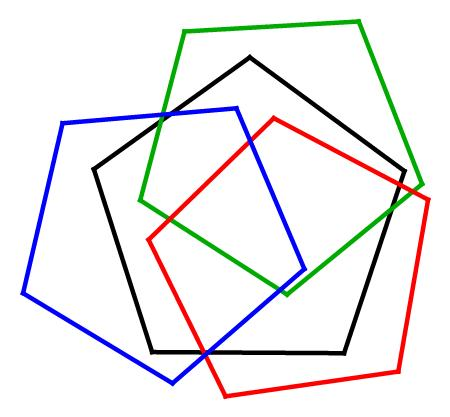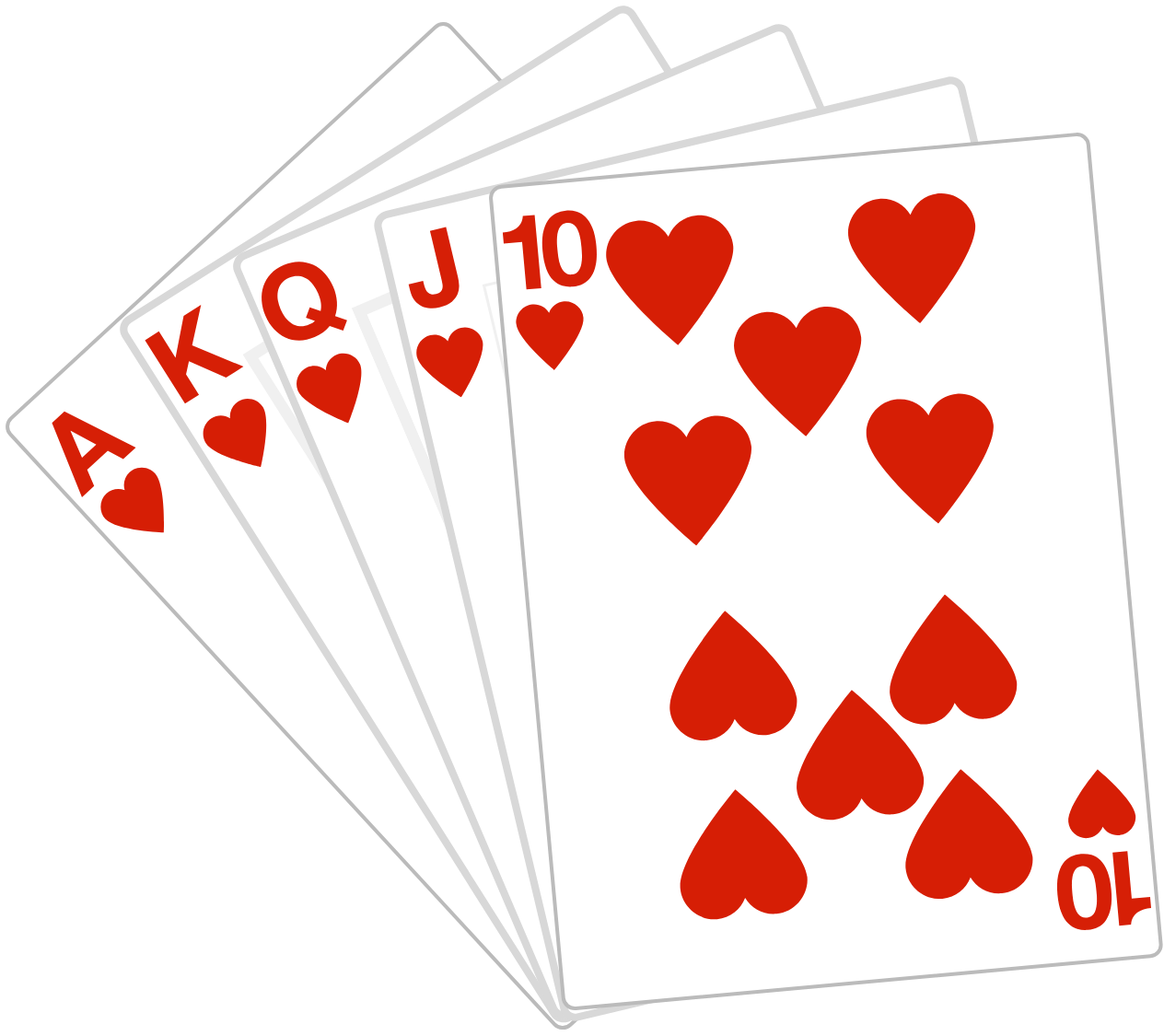# Problems of the Week

Contribute a problem

What is the last digit of the smallest positive integer $N$ that can be written as the sum of two or more consecutive positive integers in at least 2018 ways?

Bonus: Can you find $N?$

$\ m^{5}-n^{5}=16mn$

What are all the ordered pairs $(m,n)$ of integers that satisfy this equation?

Enter your answer as the total sum of all the $m$'s and $n$'s.

In this diagram, three regular pentagons (red, blue, green) of side length $1$ overlap to completely cover a fourth regular pentagon (black) of side length $s.$

What's the largest possible value of $s?$

Give your answer as $\left\lfloor 1000s \right\rfloor.$Note: You can rotate or place the pentagons however you want.

The set $S$ contains integers in the range $1$ to $50$ inclusive.

What is the largest number of elements you can have in the set such that no two numbers in the set can be multiplied by the same integer to form perfect squares?

For example, $1$ and $4$ can't both be in the set since they could each be multiplied by $9$ to form perfect squares, $9$ and $36$.

You shuffle a standard deck of cards, draw the top card, and place it on the table. You keep doing this until you can form a flush from the cards you have drawn.

What is the expected number of cards you will draw (rounded to the nearest integer)?

Note: A standard deck of cards has 52 cards in total, with 4 different suits. A flush is formed when there are 5 cards of the same suit.×